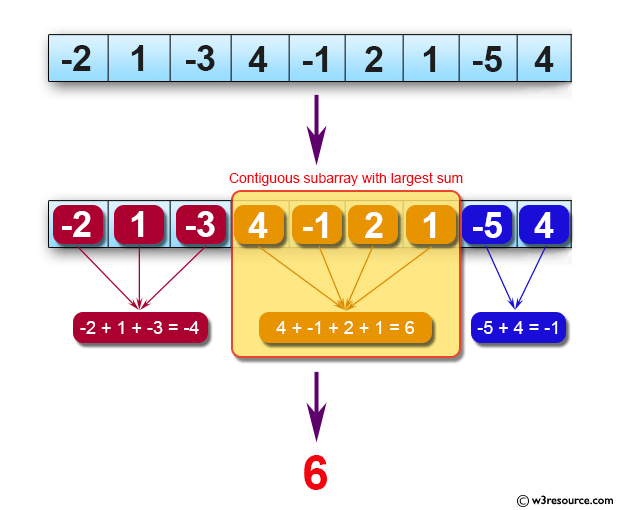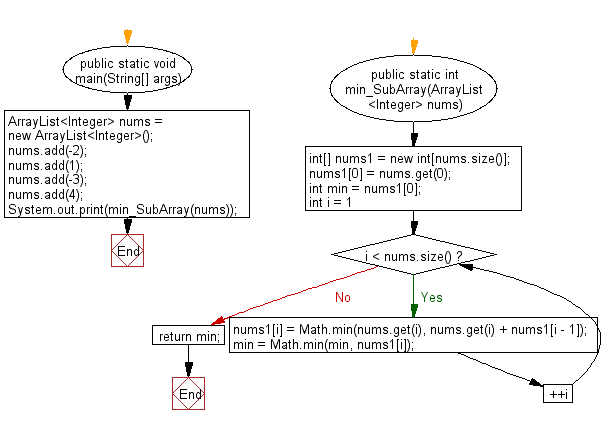﻿ Java: Find a subarray with largest sum from an array# Java Exercises: Find a contiguous subarray with largest sum from a given array of integers

## Java Basic: Exercise-122 with Solution

Write a Java program to find a contiguous subarray with largest sum from a given array of integers.
Note: In computer science, the maximum subarray problem is the task of finding the contiguous subarray within a one-dimensional array of numbers which has the largest sum. For example, for the sequence of values −2, 1, −3, 4, −1, 2, 1, −5, 4; the contiguous subarray with the largest sum is 4, −1, 2, 1, with sum 6.
The subarray should contain one integer at least.

Pictorial Presentation:Sample Solution:

Java Code:

``````public class Main {
public static void main(String[] args) {
int[] nums = {-2, 1, -3, 4, -1, 2, 1, -5, 4};
System.out.print(max_SubArray(nums));
}
public static int max_SubArray(int[] nums) {
if (nums.length < 1) {
return 0;
}
int max = nums;
int max_Begin = 0;
int max_End = 0;
int begin = 0;
int end = 0;
int sum = 0;
while (end < nums.length) {
sum += nums[end];
if (sum < 0) {
sum = 0;
begin = end + 1;
} else {
if (sum > max) {
max = sum;
max_Begin = begin;
max_End = end;
}
}
end++;
}
return max;
}
}
```
```

Sample Output:

```6
```

Flowchart:Java Code Editor:

What is the difficulty level of this exercise?

Test your Programming skills with w3resource's quiz.

﻿

## Java: Tips of the Day

countOccurrences

Counts the occurrences of a value in an array.

Use Arrays.stream().filter().count() to count total number of values that equals the specified value.

```public static long countOccurrences(int[] numbers, int value) {
return Arrays.stream(numbers)
.filter(number -> number == value)
.count();
}
```

Ref: https://bit.ly/3kCAgLb